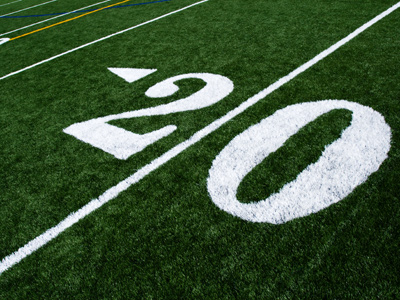Which numbers are less than 20?

# Level 7-8 Algebra - Inequalities

So you are familiar with the symbols used in KS3 Maths - plus (+), minus (-), times (x), divide (÷) and equals (=). You'll also have come across some more unusual ones like squared (2), cubed (3), square root (√) and cube root (∛). But there are some other symbols which you are (hopefully!) aware of which you'll see a lot of in algebra - the signs for inequalities: less than (<), greater than (>), less than or equal to (≤) and greater than or equal to (≥).

This quiz deals with the relationships of one item to another. We use the inequalities symbols to show how numbers (often variables) compare to one another. First we look at the symbols involved, just to make sure you recognise each one. After that you'll find some algebraic questions you will undoubtedly enjoy answering!

Take your time with the following 10 questions. You may think this quiz is very easy, but don't be fooled! Over confidence can lead to silly mistakes for the unwary. Good luck!

1.
< is the sign that means what?
Less than
Greater than
Less than or equal to
Greater than or equal to
This might help you remember which way the less than sign points: Less = Left (pointing)
2.
> is the sign that means what?
Less than
Greater than
Less than or equal to
Greater than or equal to
The small end of the sign always points to the smaller number
3.
≤ is the sign that means what?
Less than
Greater than
Less than or equal to
Greater than or equal to
Numbers that are < 6 are 5, 4, 3 etc; numbers that are ≤ 6 are 6, 5, 4, etc. Can you see the difference? If not then look again because it is important that you understand the difference between these signs
4.
≥ is the sign that means what?
Less than
Greater than
Less than or equal to
Greater than or equal to
Remember, the arrow always points to the smaller number
5.
If x > 3, what is the smallest integer that x can be?
2
3
4
5
x must be greater than three and the next integer after three is four
6.
If x ≥ 19, which of the following numbers is not possible for the value of x?
18
19
20
21
18 is less than 19
7.
If 4x + 3 < 15, which of the following expressions are incorrect?
4x < 15 - 3
4x < 12
x < 3
x > 3
When a number crosses over the < or > signs then it changes from positive to negative or vice versa
8.
If 4x - 7 < 17, which of the following expressions are incorrect?
4x < 17 + 7
4x < 24
x > 6
x < 6
Values for x are less than 6
9.
If 16x - 5 > 59, which of the following is correct?
16x > 64
16x > 59
16x < 59
16x < 64
It is extremely easy to mix-up < and > so be careful!
10.
If 9x + 40 > 140 which of these is correct?
9x - 40 > 140
9x > 100
9x < 100
x > 20
If 9x + 40 > 140 then 9x must be > 100